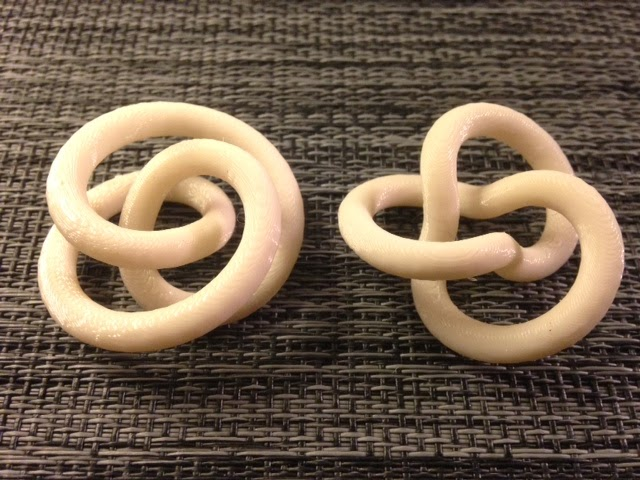# Day 150 – Trefoil torus knots## Day 150 – Trefoil torus knots

Day 150 – Trefoil torus knots 640 480 mathgrrl

For the next four days we’ll be posting various mathematically interesting models of the trefoil knot. Mathematicians consider two knotted forms to be equivalent if one can be transformed into the other by stretching and moving in space, without cutting or joining. The way a knot sits in space is known as its conformation. Two of the best-known shapes for the trefoil knot are the T(2,3) and T(3,2) torus knot conformations:

STL file for T(2,3): http://www.geekhaus.com/makerhome/day150_trefoil_torus23_40_25_3.stl
STL file for T(3,2): http://www.geekhaus.com/makerhome/day150_trefoil_torus32_40_25_3.stl

Settings: MakerWare .3mm/low in 20-25 minutes per knot, with the custom slicing profile from Day 110.

Technical notes, math flavor: Torus knots are knots that can be wrapped around a torus (inner-tube or donut shape) without any self-intersections. The torus knot T(p,q) wraps p times around the torus like a clock at the same time that it wraps q times around the handle of the torus. The knot T(p,q) is always equivalent to the knot T(q,p), even though the two conformations can at first glance appear to be quite different. In the picture below from Paul Aspinwall at Duke University, the torus knot T(3,5)=T(5,3) wraps 3 times around the outside and 5 times around the handle.

The parametric equations for a torus with handle radius a and large radius c are (see Wolfram):

```x = (c + a cos v) cos u
y = (c + a cos v) sin u
z = a sin v```

If we substitute u=pt and v=qt then as t runs from 0 to 2휋 we obtain a curve that traces the T(p,q) torus knot around the surface of the torus (and if p and q are reversed then we get the T(q,p) torus knot).

// mathgrrl parametric knots – torus trefoils
// tubify module based on tube module from kitwallace

// http://www.thingiverse.com/thing:230557/
// remove comments for the one you want to compile

/*
// trefoil as the torus knot T(2,3)
// http://mathworld.wolfram.com/Torus.html
// take parameterization of torus (u,v)->R^3
// and let u=2t, v=3t
// scaled to 40mm before tubifying
function f(t) =
[ 3.9*(3+1.6*cos(2*t))*cos(3*t),
3.9*(3+1.6*cos(2*t))*sin(3*t),
3.9*(1.6*sin(2*t))
];
// create the knot with given radius and step
tubify(2.5, 12, 360);
*/

/*
// trefoil as the torus knot T(3,2)
// http://mathworld.wolfram.com/Torus.html
// take parameterization of torus (u,v)->R^3
// and let u=2t, v=3t
// scaled to 40mm before tubifying
function f(t) =
[ 3.9*(3+1.6*cos(3*t))*cos(2*t),
3.9*(3+1.6*cos(3*t))*sin(2*t),
3.9*(1.6*sin(3*t))
];
// create the knot with given radius and step
tubify(2.5, 3, 360);
*/

module tubify(r, step, end) {
for (t=[0: step: end+step]) {
hull() {
translate(f(t)) sphere(r);
translate(f(t+step)) sphere(r);
}
}
};

——————

As an Amazon Associate we earn from qualifying purchases, so if you’ve got something you need to pick up anyway, going to Amazon through this linkwill help us keep Hacktastic running. Thanks! :)

•nice Torii ! must be so nice to have your own printer ( he says whistfully)

I put a Torus customizer on Thingiverse http://www.thingiverse.com/thing:231727 – it only supports the t(u,v) parameters but it does include other shape parameters

re tubify, replacing cones with hulled cones is certainly faster than hulled spheres (but they need orientating) – the mailing list has got stuck into this problem and developments afoot look very promising, albeit that they dont deal with self-intersecting curves

•okay, i think the file is uploaded correctly now, sorry about that! i tried to write a cylinders-oriented-by-directional-derivative type of thing but it was so. very. slow. and i couldn't get it to work out. and in the corners i would have had to add spheres anyway. i'm definitely interested in anything people are working on in this capacity. what kind of mailing list are you on and is it private or could i possibly join it?

•The rollingknot code with cones is now on github https://github.com/KitWallace/openscad/blob/master/rollingknot.scad – it seemed to me more than twice as fast as spheres.

The github mailing list is http://forum.openscad.org/ – this is where the real openscad gurus hang out

•As noted elsewhere, you have the opposite usage of p and q in T(p, q) as is commonly used, and as used in the Wolfram Mathworld source to which you give a link

•Yeah, since they are the same knot I play a bit fast and loose with that

Share